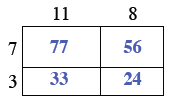Home > AC > Chapter 5 > Lesson 5.1.1 > Problem5-4

5-4.

For the entire rectangle at right, find the area of each part and then find the area of the whole. Homework Help ✎

First, find the area of each individual box.Add all of the areas together to get the entire area of the rectangle.

$190$ square units

 $11$ $8$ $7$ $3$